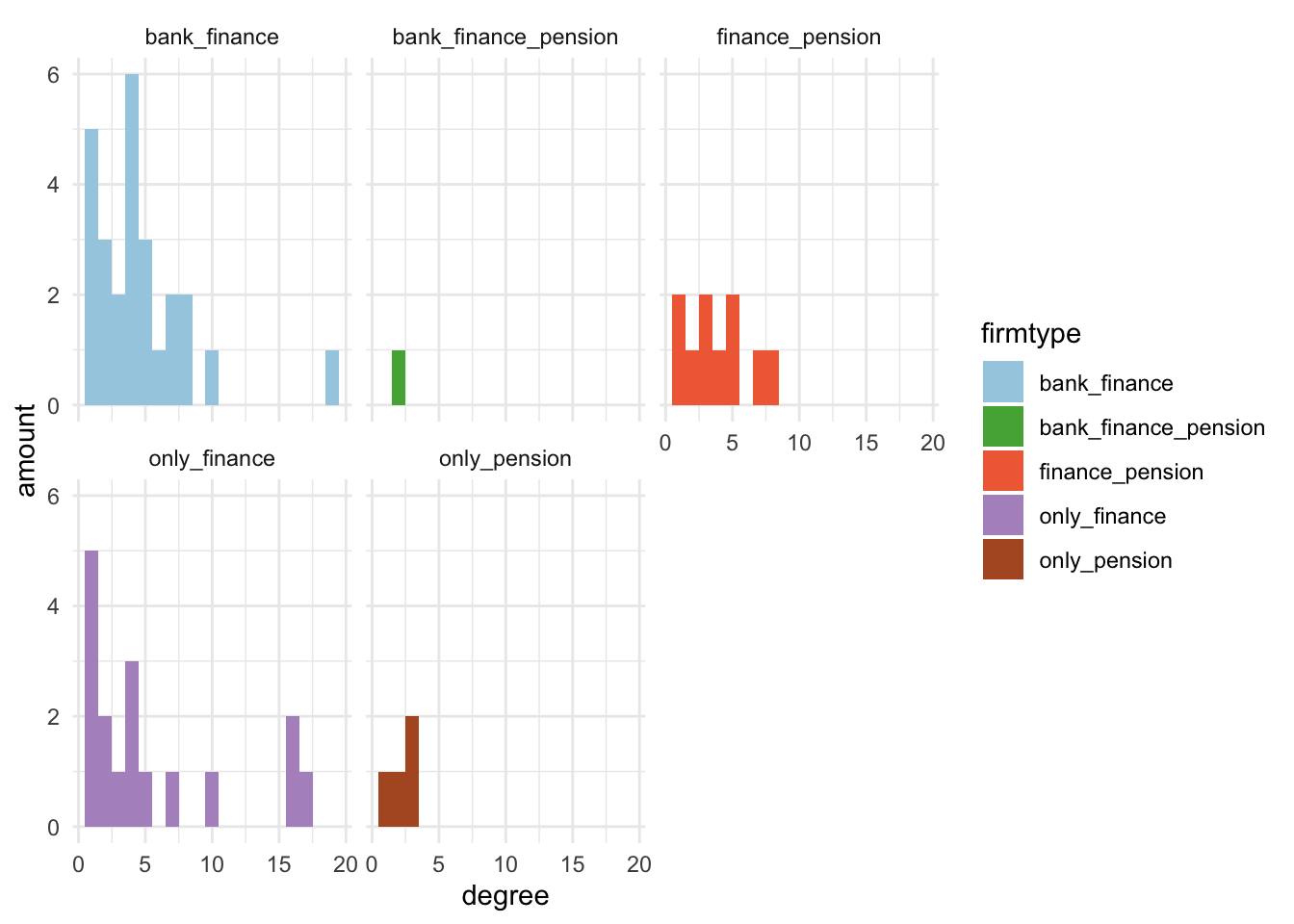# Session 7 - Visualization## Session 7 - Network visualization #

This is the last session of this course. During this session, we will further sharpen your visualization skills using the `ggraph` package. We will cover topics such as adding graph attributes, scaling and tweaking network visualizations, modifying the legend, and adding a title. With the `ggraph` package, you will have a range of options to customize the visual output and create high-quality network visualizations in pdf format.

``````#setting the working directory
setwd("")

library(tidyverse)
library(data.table)
library(ggraph)
library(igraph)
library(graphlayouts)
# for cleaning functions in orbis
source("r/custom_functions.R")
library(purrr)
library(RColorBrewer)
library(writexl) # if error, then install.packages("writexl")

# Subsetting den17 dataset by tags ----------------------------------------

# subset of corporations that have a valid cvr affiliation
den1 <-
den %>% filter(sector %in% "Corporations")

# selecting finance tag
den2 <- has.tags(den1, c("Finance", "Banks", "Pensions"), result = "den")

# creating an incidence matrix
incidence <- xtabs(formula = ~ name + affiliation,
data = den2,
sparse = TRUE)

# adjacency matrix for chosing corporations

# one-mode graph for corporations
simplify(remove.multiple = TRUE, remove.loops = TRUE)

# quick visualization
autograph(gr)

# components & plotting ---------------------------------------------------

# What are the components?
complist <- components(gr)

# Decompose graph
comps <- decompose.graph(gr)

# Create an index
index <-
table(complist\$membership) %>%
as_tibble(.name_repair = make.names) %>%
arrange(desc(n)) %>%
mutate(X = as.numeric(X)) %>%
pull(1)

# Select the largest component
comp1 <- comps[[index]]
``````

## 7.1 Graph attributes #

In the first part of this session we add some graph attributes that are later visualized. First, I am adding an attribute called `firmtype` and second, other node centrality attributes are added. Now, the `has.tags()` function is used to find a subset of the network based on affiliation. This function takes three arguments: the network data, the tag name, and the result type (i.e., whether to return the affiliation or the name of the nodes). This creates subsets of the `den17` data set that are tagged with “Finance,” “Banks,” and “Pensions.”

``````###### 1. ADD SECTOR ######

# finding subset for only the biggest component
den3 <- den2 %>% filter(affiliation %in% V(comp1)\$name)

# finding those with only a tag for finance, pension or banks
finance <- has.tags(den3, "Finance", result = "affil")
``````
``````##         Matched positions
## Finance               558
##
##
``````
``````bank <- has.tags(den3, "Banks", result = "affil")
``````
``````##       Matched positions
## Banks               272
##
##
``````
``````pension <- has.tags(den3, "Pensions", result = "affil")
``````
``````##          Matched positions
## Pensions               180
##
##
``````

Next, the `rbind()` function is used to combine these subsets into a single tibble with two columns: “name” and “tag.” Then, `xtabs()` is used to cross-tabulate the tibble into a sparse matrix format, which is then converted into a tibble format using the `as_tibble()` function.

``````# making a tibble
a1 <- rbind(
tibble(name = finance, tag = "finance"),
tibble(name = bank, tag = "bank"),
tibble(name = pension, tag = "pension")
)

# cross tabulating
a2 <- xtabs(formula = ~ name + tag, data = a1, sparse = TRUE)

# change matrix to tibble format
a2 <- a2 %>% as.matrix() %>% as_tibble(rownames = "name")
``````

Following this, the code uses a series of `filter()` and `pull()` functions to create separate vectors of firm names based on their sector (e.g., `only_bank`, `only_finance`, `bank_finance`, etc.). The `NA` firmtype attribute is added to the network using `V(comp1)\$firmtype <- NA`. The firmtype attribute is then assigned to each node based on its name, using a series of `which()` statements and the `<-` operator.

``````# make vectors with different names depending on the possible firm types
only_bank <- a2 %>% filter(bank==1 & finance==0 & pension ==0) %>% pull(name)
only_finance <- a2 %>% filter(bank==0 & finance==1 & pension ==0) %>% pull(name)
only_pension <- a2 %>% filter(bank==0 & finance==0 & pension ==1) %>% pull(name)
bank_finance <- a2 %>% filter(bank==1 & finance==1 & pension ==0) %>% pull(name)
bank_pension <- a2 %>% filter(bank==1 & finance==0 & pension ==1) %>% pull(name)
finance_pension <- a2 %>% filter(bank==0 & finance==1 & pension ==1) %>% pull(name)
bank_finance_pension <- a2 %>% filter(bank==1 & finance==1 & pension ==1) %>% pull(name)

# make an empty network attribute
V(comp1)\$firmtype <- NA

# rename for reach firm type
V(comp1)\$firmtype[which(V(comp1)\$name %in% only_bank)] <- "only_bank"
V(comp1)\$firmtype[which(V(comp1)\$name %in% only_finance)] <- "only_finance"
V(comp1)\$firmtype[which(V(comp1)\$name %in% only_pension)] <- "only_pension"
V(comp1)\$firmtype[which(V(comp1)\$name %in% bank_finance)] <- "bank_finance"
V(comp1)\$firmtype[which(V(comp1)\$name %in% bank_pension)] <- "bank_pension"
V(comp1)\$firmtype[which(V(comp1)\$name %in% finance_pension)] <- "finance_pension"
V(comp1)\$firmtype[which(V(comp1)\$name %in% bank_finance_pension)] <- "bank_finance_pension"

# Look at the result
V(comp1)\$firmtype
``````
``````##   "only_finance"         "finance_pension"      "bank_finance"
##   "only_pension"         "only_finance"         "bank_finance"
##   "only_finance"         "finance_pension"      "only_finance"
##  "bank_finance"         "bank_finance"         "bank_finance"
##  "bank_finance"         "bank_finance"         "only_finance"
##  "bank_finance"         "only_pension"         "only_finance"
##  "finance_pension"      "bank_finance"         "bank_finance"
##  "bank_finance"         "bank_finance"         "only_finance"
##  "bank_finance"         "only_pension"         "finance_pension"
##  "bank_finance"         "finance_pension"      "only_finance"
##  "only_finance"         "bank_finance"         "bank_finance_pension"
##  "bank_finance"         "bank_finance"         "finance_pension"
##  "only_pension"         "finance_pension"      "finance_pension"
##  "only_finance"         "only_finance"         "only_finance"
##  "bank_finance"         "finance_pension"      "only_finance"
##  "only_finance"         "finance_pension"      "bank_finance"
##  "only_finance"         "bank_finance"         "bank_finance"
##  "bank_finance"         "bank_finance"         "bank_finance"
##  "bank_finance"         "only_finance"         "only_finance"
##  "bank_finance"
``````

Second, the degree, betweenness and closeness centrality measures are calculated using the `degree()` `betweenness()` and `closeness()` functions, respectively. These centrality measures are then added to the network using the `\$` operator and assigned to each node based on its name.

``````###### 2. ADD CENTRALITY ######

# degree
V(comp1)\$degree <- degree(comp1, mode = "all")

#betweenness
V(comp1)\$betweenness <- betweenness(comp1, directed = FALSE)

# closeness
V(comp1)\$closeness <-  closeness(comp1, mode = "all")
``````

Now, we are ready to visualize these attributes.

## 7.2 Graph visualizations #

In this section of the code, the graph attributes that were created earlier are used to visualize the network. The visualization is done using the `ggraph` package, which is a package for creating network visualizations in R.

The first line of code creates a `ggraph` object with a Fruchterman-Reingold (`fr)` layout. This layout places nodes that are connected by edges close to each other and nodes that are not connected far from each other. The `geom_edge_link0` function is used to draw the edges of the graph. The `width` argument sets the width of the edges and the `alpha` argument sets how visible the edges are.

Next, the `geom_node_point` function is used to draw the nodes of the graph. The `color` aesthetic is set to `firmtype`, which was defined earlier as a graph attribute that categorizes each node based on the type of firm it represents. The `size` aesthetic is set to `betweenness`, which was also defined earlier as a graph attribute that measures the importance of each node based on how many shortest paths between other nodes it lies on. The `alpha` argument sets the transparency of the nodes.

The `geom_node_label` function is used to add labels to the nodes. The `filter` argument selects only nodes with a `betweenness` value greater than 250. The `color` aesthetic is set to `firmtype`, which categorizes the nodes. The `label` aesthetic is set to `name`, which is the name of each node. The `size` argument sets the font size of the labels, and the `repel` argument sets whether or not the labels should overlap with each other.

Finally, the `theme_graph` function is used to apply a theme to the visualization.

``````# visualization with node color as firmtype and node size as degree
v1 <- comp1 %>%
ggraph(
#layout could be one of the above
layout = "fr") +
# width of the line
width=0.5,
# how visible the line is (from 0-1)
alpha=0.4,
color = "grey60") +
geom_node_point(
# different for every node
aes(color=firmtype, size = betweenness),
alpha = 0.95) +
geom_node_label(
aes(filter=betweenness>250, color=firmtype, label=name),
size=2,
repel=TRUE) +
theme_graph()

v1
``````
``````## Warning: Using the `size` aesthetic in this geom was deprecated in ggplot2 3.4.0.
## ℹ Please use `linewidth` in the `default_aes` field and elsewhere instead.
## This warning is displayed once every 8 hours.
## Call `lifecycle::last_lifecycle_warnings()` to see where this warning was
## generated.
``````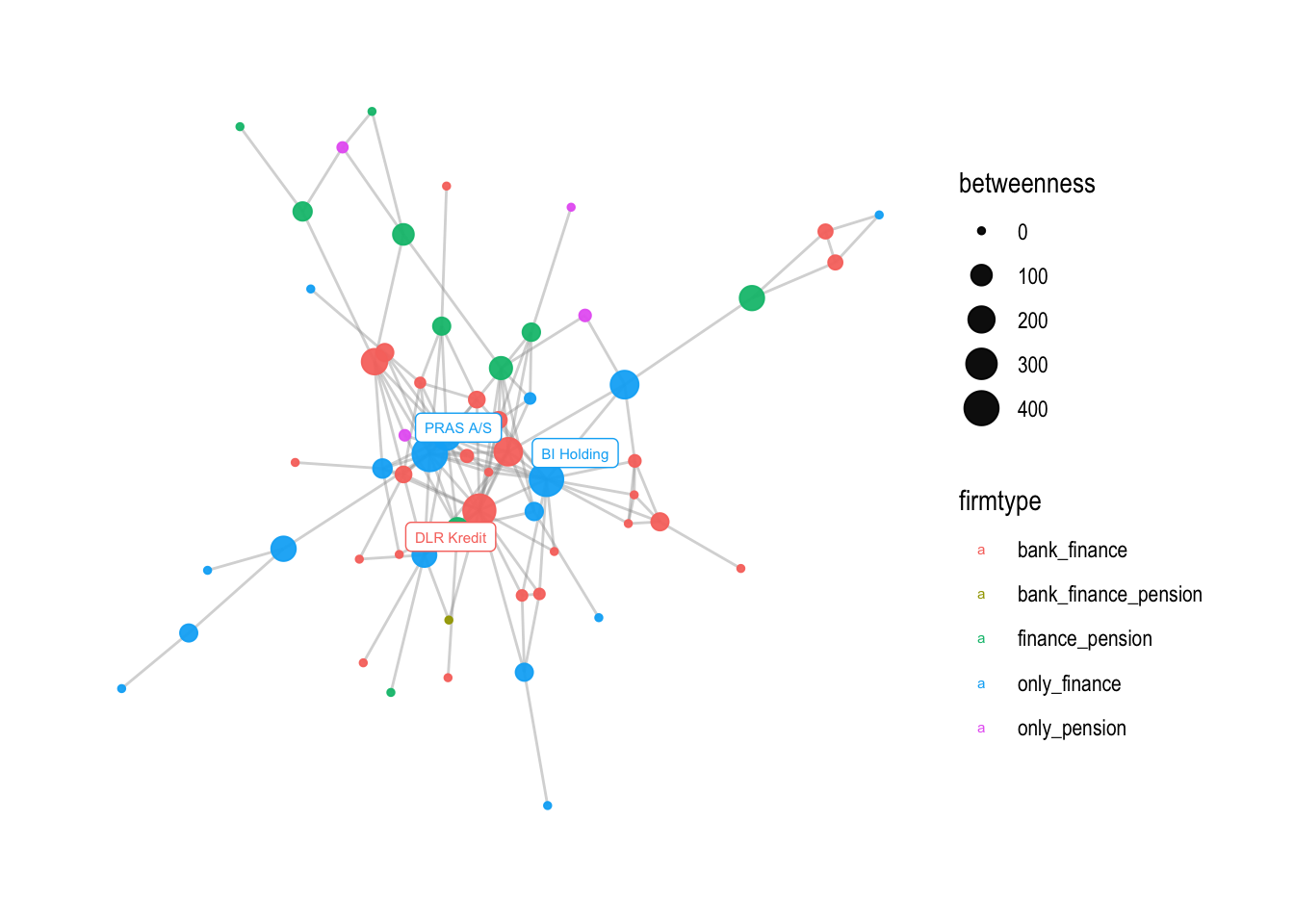In the next section of the code, `v2` is created by adding a small black dot to the location of the labeled nodes using `geom_node_point`. This helps to make the labeled nodes more visible.

``````v2 <- v1 +
# add a little dark point to where the labelled nodes are
geom_node_point(aes(filter=betweenness>250), color = "black", size =0.5)

v2
``````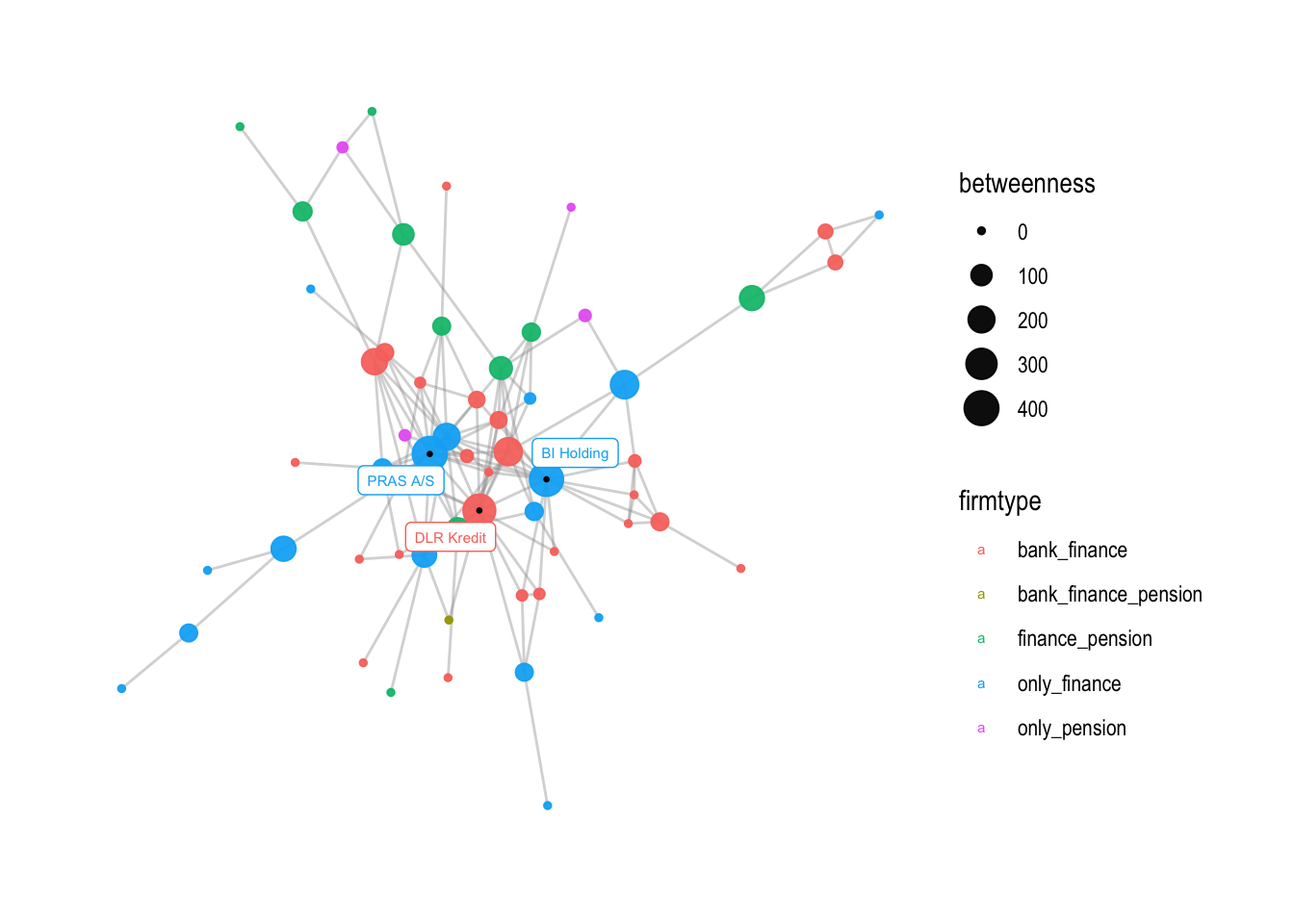`v3` shows how the range of the node sizes can be changed using `scale_size_continuous`. The `range` argument sets the minimum and maximum node sizes. In this case, the minimum size is set to 1 and the maximum size is set to 7. The `breaks` argument sets the values at which ticks are placed on the legend for the node size. In this case, ticks are placed at intervals of 50 between 0 and 400.

``````# if you want to change the size range of nodes, you can use this command
v3 <- v2 + scale_size_continuous(range = c(1,7))

v3
``````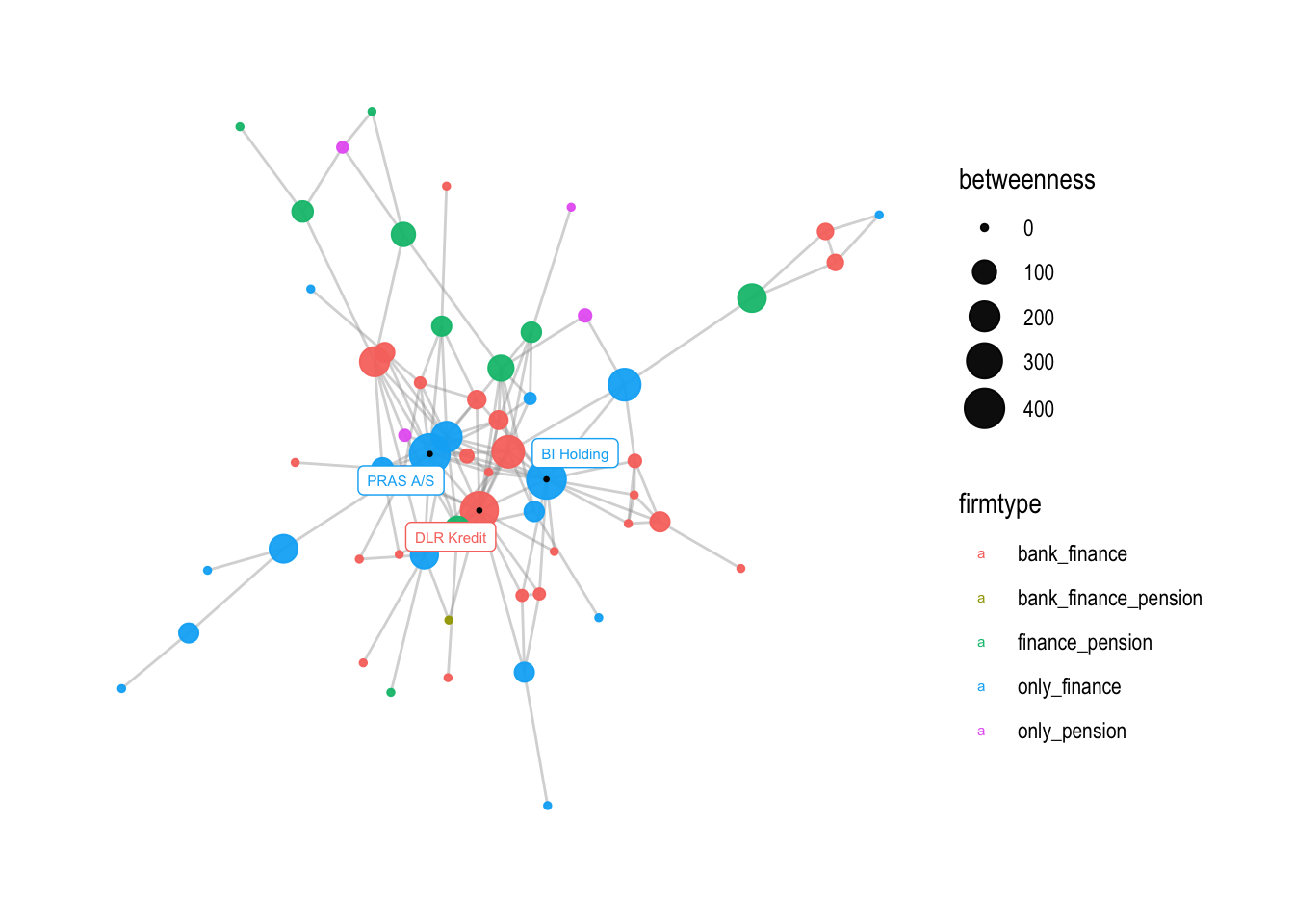``````# you can also change the breaks
v3 + scale_size_continuous(range = c(1,7),
breaks = c(0,50,100,150,200,250,300,350,400))
``````
``````## Scale for size is already present.
## Adding another scale for size, which will replace the existing scale.
``````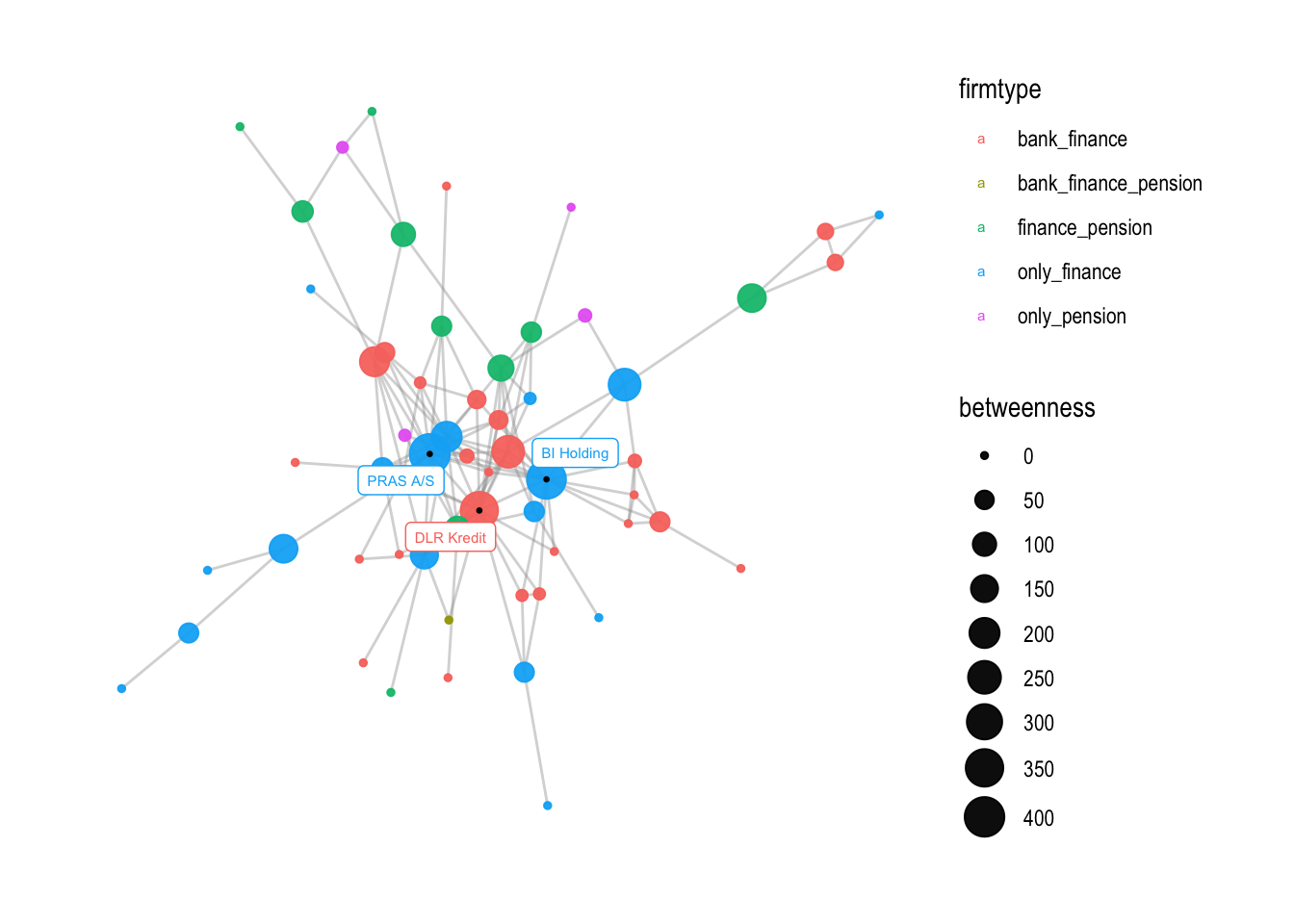If you want, you can also change the size of the legend with the following command:

``````# changing the size of the firmtype points in the legend
v3 + guides(color = guide_legend(override.aes = list(size = 3)))
``````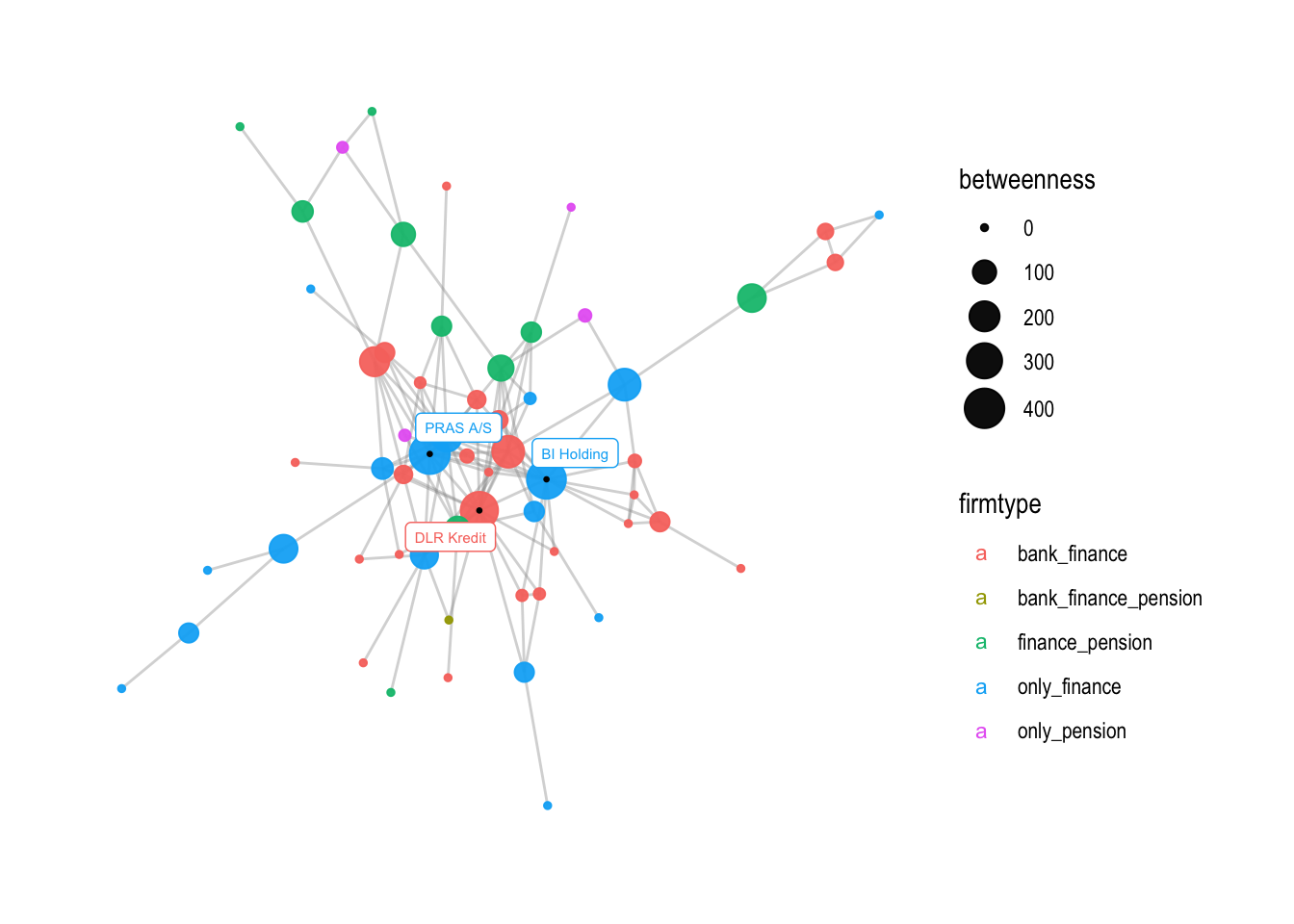The next step is to change the names on the legend. This can be achieved using the `labs()` function, which is used to modify the titles and axis labels of a plot. In this case, we change the size and color legend titles to “Betweenness centrality” and “Firmtype”, respectively:

``````# change the names on the legend
v4 <- v3 + labs(
size="Betweenness centrality",
color="Firmtype")

v4
``````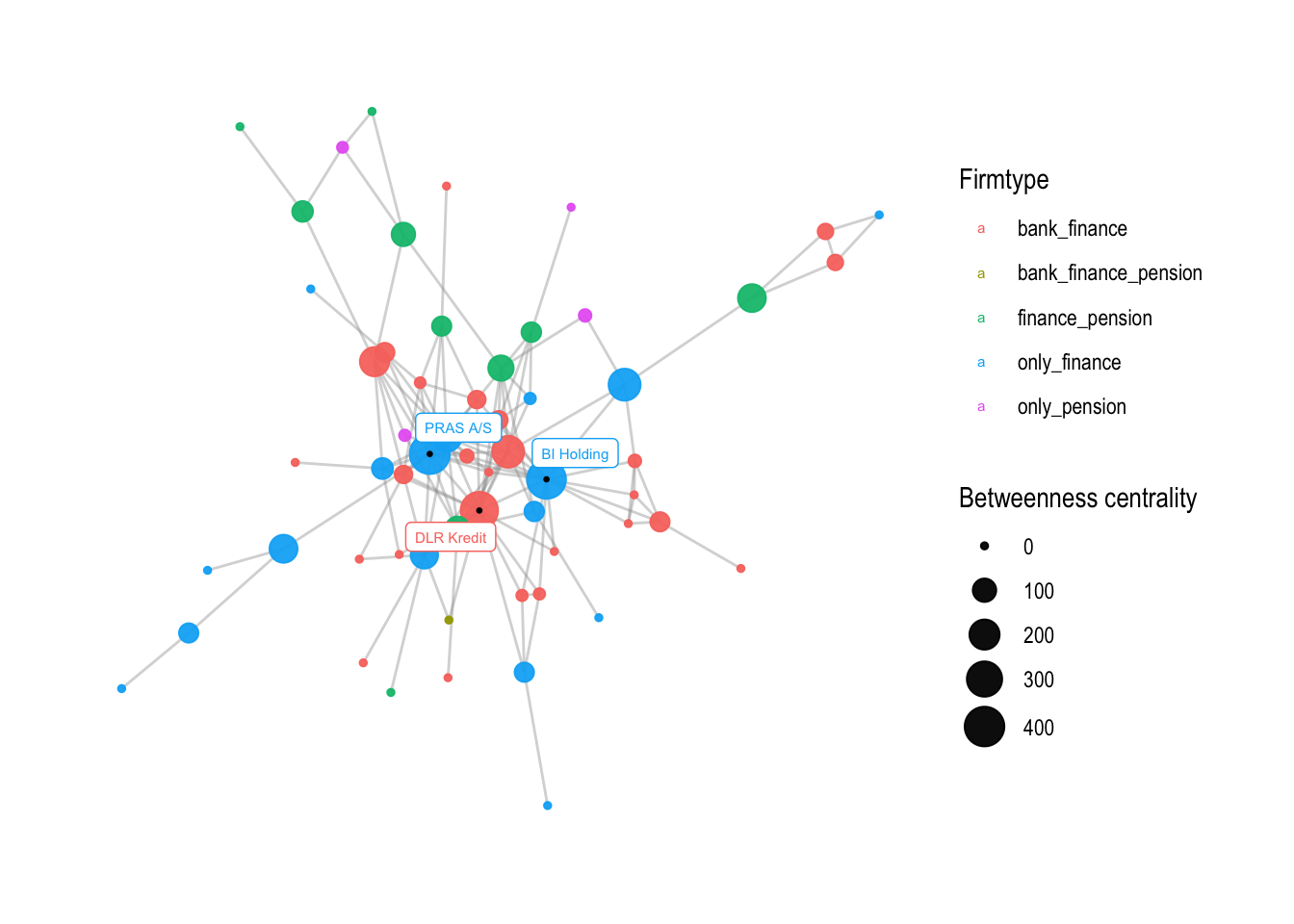The resulting plot `v4` will have modified legend titles.

Next, we change legend names and adapt colors, choosing a different color palette. To retrieve a specific color scheme, we can use the `display.brewer.all()` function to view a list of color palettes available. Here, we use the “Paired” palette from the `RColorBrewer` package, which contains 12 distinct colors. Since there are five unique `firmtype` values in our data, we need to generate five colors from the palette.

This can be achieved using the `colorRampPalette()` function:

``````# choosing different color palette
number <- V(comp1)\$firmtype %>% unique() %>% length()

# To retrieve a specific color sheme:
display.brewer.all()
``````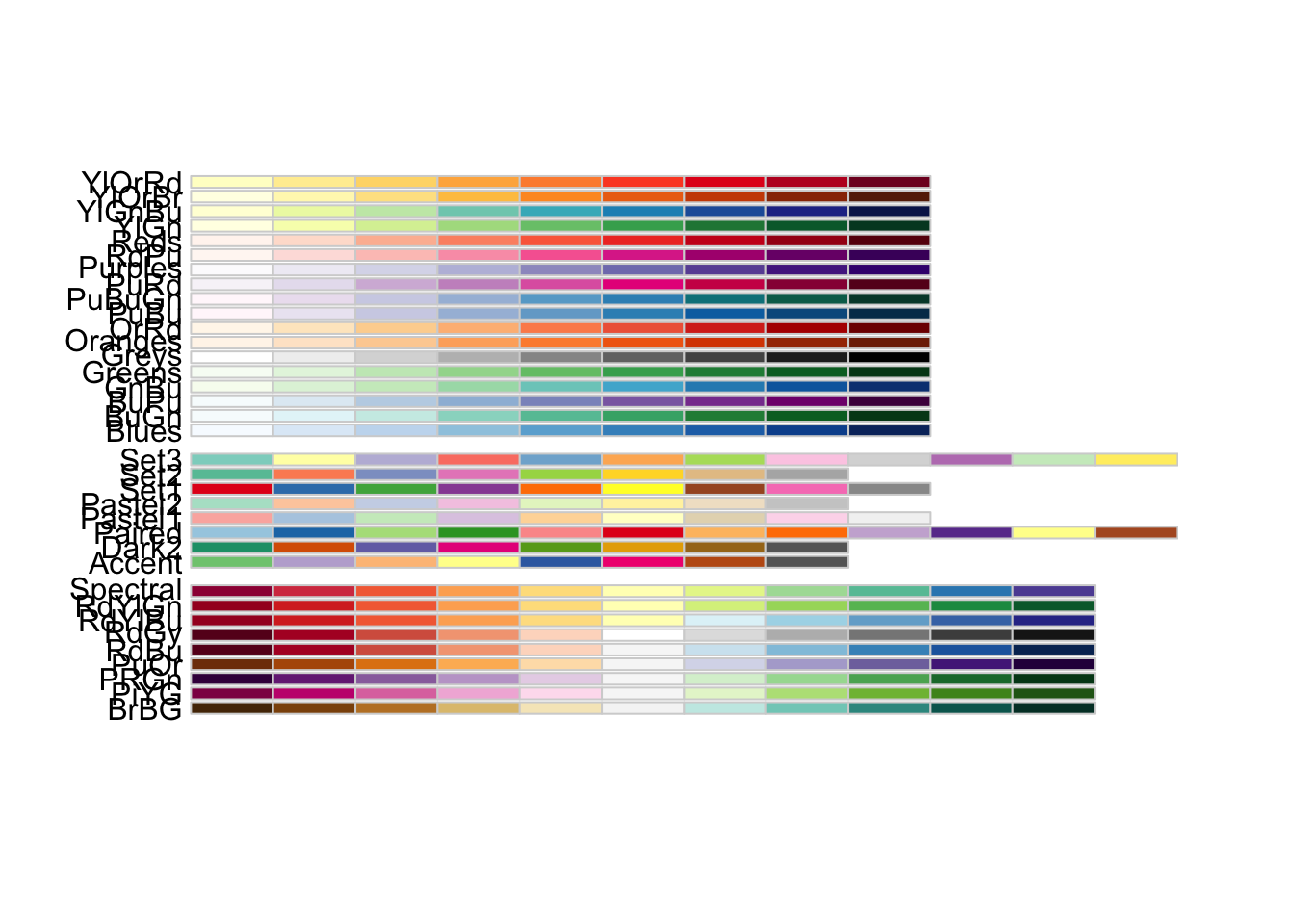``````my_colors <- colorRampPalette(brewer.pal(12, name = "Paired"))(number)
``````

The resulting `my_colors` vector will contain five colors generated from the “Paired” palette.

We can then apply these colors to the plot using the `scale_color_manual()` function, which allows us to manually set the colors of legend values. We also specify the breaks and labels for each `firmtype` value using the `breaks` and `labels` arguments:

``````# change not only the values, but also the labels
v5 <- v4 +
scale_color_manual(values = my_colors,
breaks=c('only_finance', 'only_pension', 'bank_finance', 'finance_pension', 'bank_finance_pension'),
labels=c('Finance', 'Pension', 'Bank & Pension', 'Finance og Pension', 'Bank, Finance\n& Pension'))

v5
``````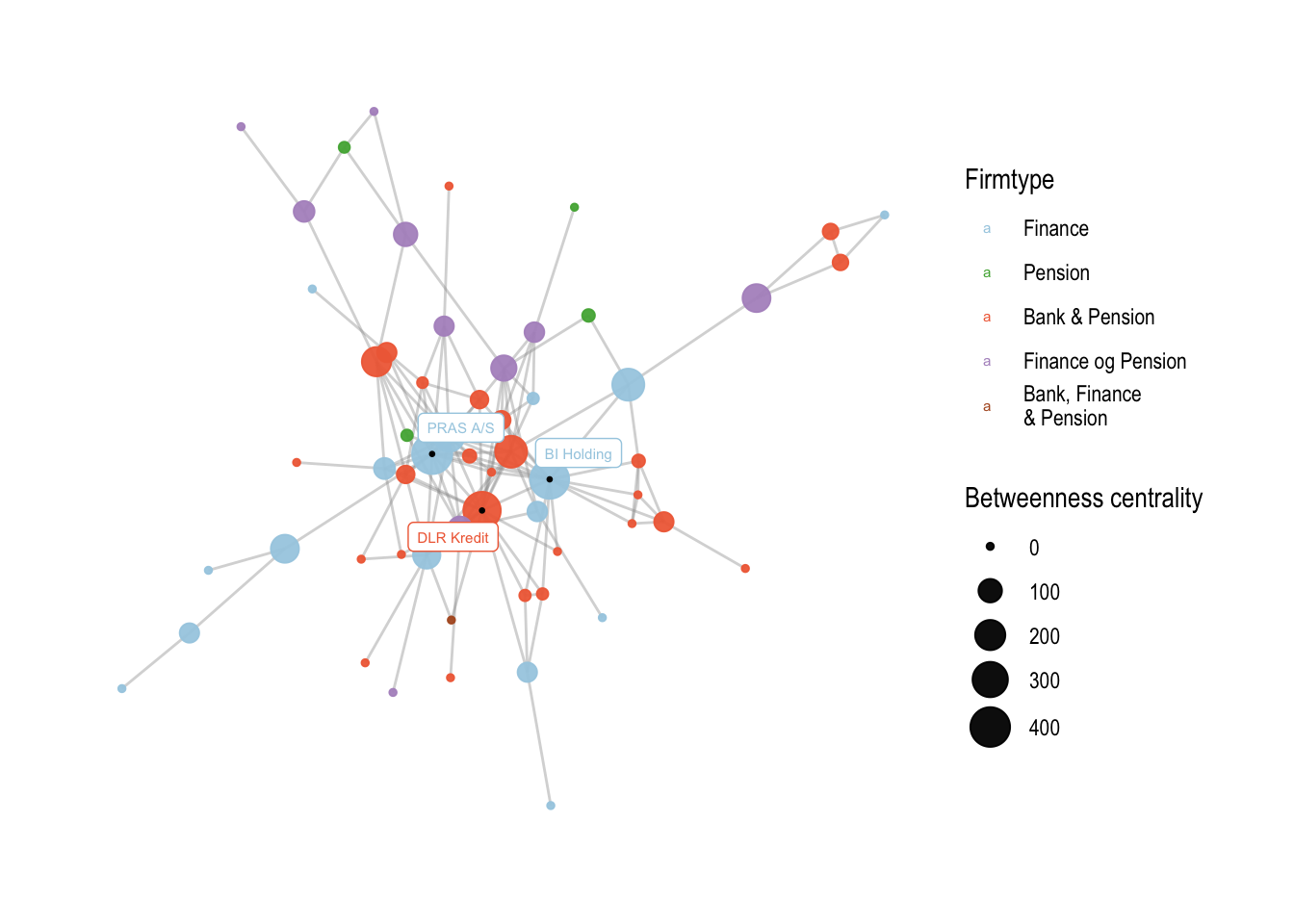The resulting plot `v5` will have modified legend values with custom colors and labels.

Finally, we add a title and a subtitle to the plot using the `labs()` function:

``````# add a title and a subtitle
v6 <- v5 + labs(title = "Figure 1: Corporate interlocks in the financial industry",
subtitle = "Something")

v6
``````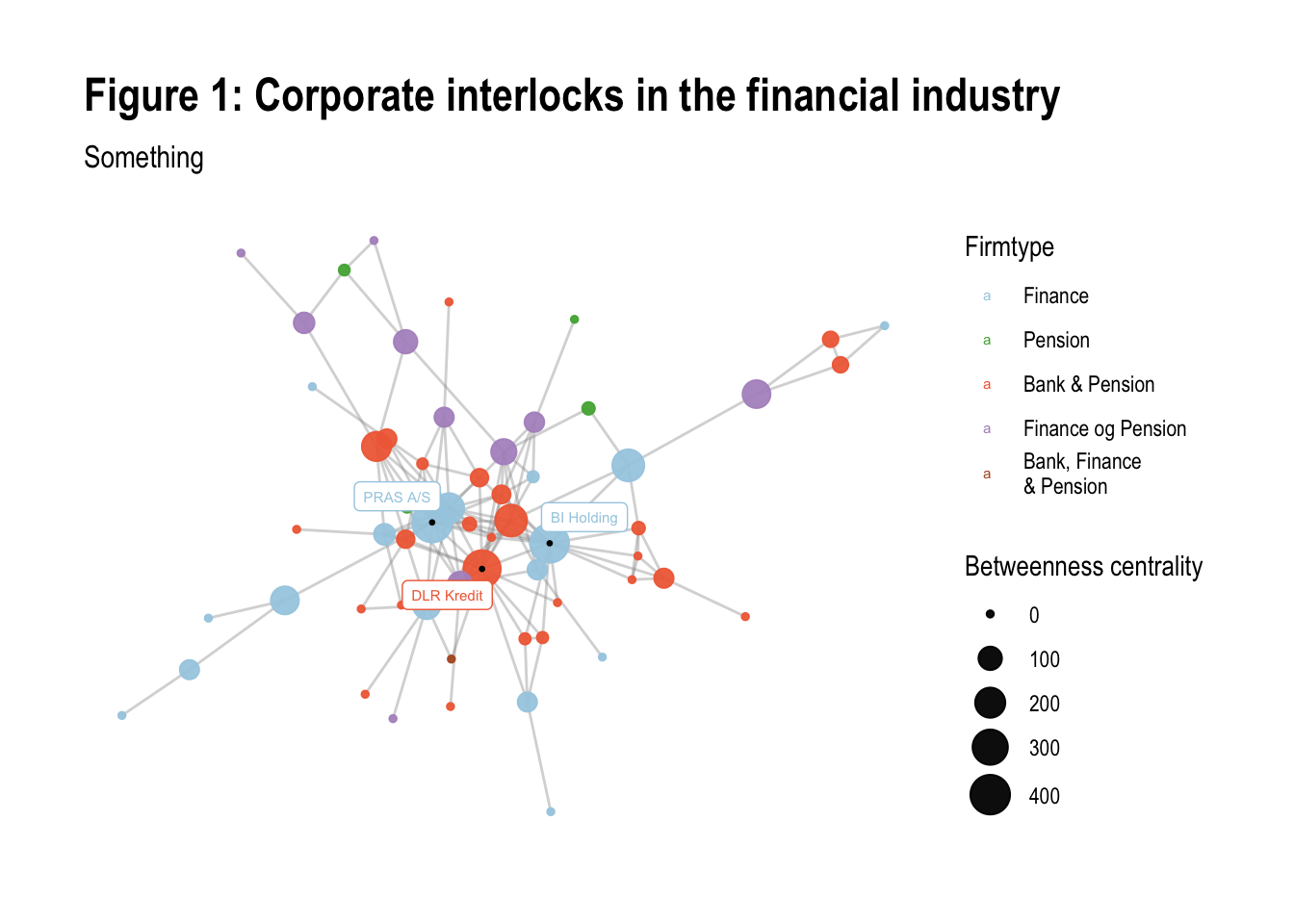The resulting plot `v6` will have a title and a subtitle.

### A second example #

In this part of the code, the focus is on creating a graph that visualizes the relationship between nodes based on two different centrality measures: closeness and betweenness.

The first step is to create a base graph using the `comp1` data frame and the `ggraph` function. This time, the `layout` parameter is set to “graphopt”, which means that the graph is generated using a force-directed layout algorithm that tries to minimize the overlap between nodes and edges.

Next, the `geom_edge_link0` function is used to draw the edges between the nodes. The `width` and `alpha` parameters control the thickness and transparency of the edges, respectively.

The `geom_node_point` function is used to draw the nodes. The `color` parameter is set to `closeness`, which means that the nodes are colored based on their closeness centrality scores. The `size` parameter is set to `betweenness`, which means that the size of the nodes is proportional to their betweenness centrality scores.

``````# Another example with closeness (and a gradient measure)

# base graph
p1 <- comp1 %>%
ggraph(layout = "graphopt") +
geom_node_point(aes(color=closeness, size=betweenness)) +
theme_graph()
``````

After setting the base graph, the `scale_size_continuous` function is used to adjust the size range of the nodes. In this case, the nodes are scaled to a range of 2 to 10.

``````# adding a size scalling argument
p1 <-  p1 + scale_size_continuous(range=c(2, 10))
``````

The next step is to change the gradient color of the nodes. Two options are shown: `scale_color_viridis` and `scale_color_gradient2`. `scale_color_viridis` is a function that provides a pre-defined gradient color scheme called “viridis”. `scale_color_gradient2` allows you to create your own gradient color scheme by specifying the low, mid, and high colors.

``````# changing the gradient color: viridis is a good option
p1 + scale_color_viridis()
``````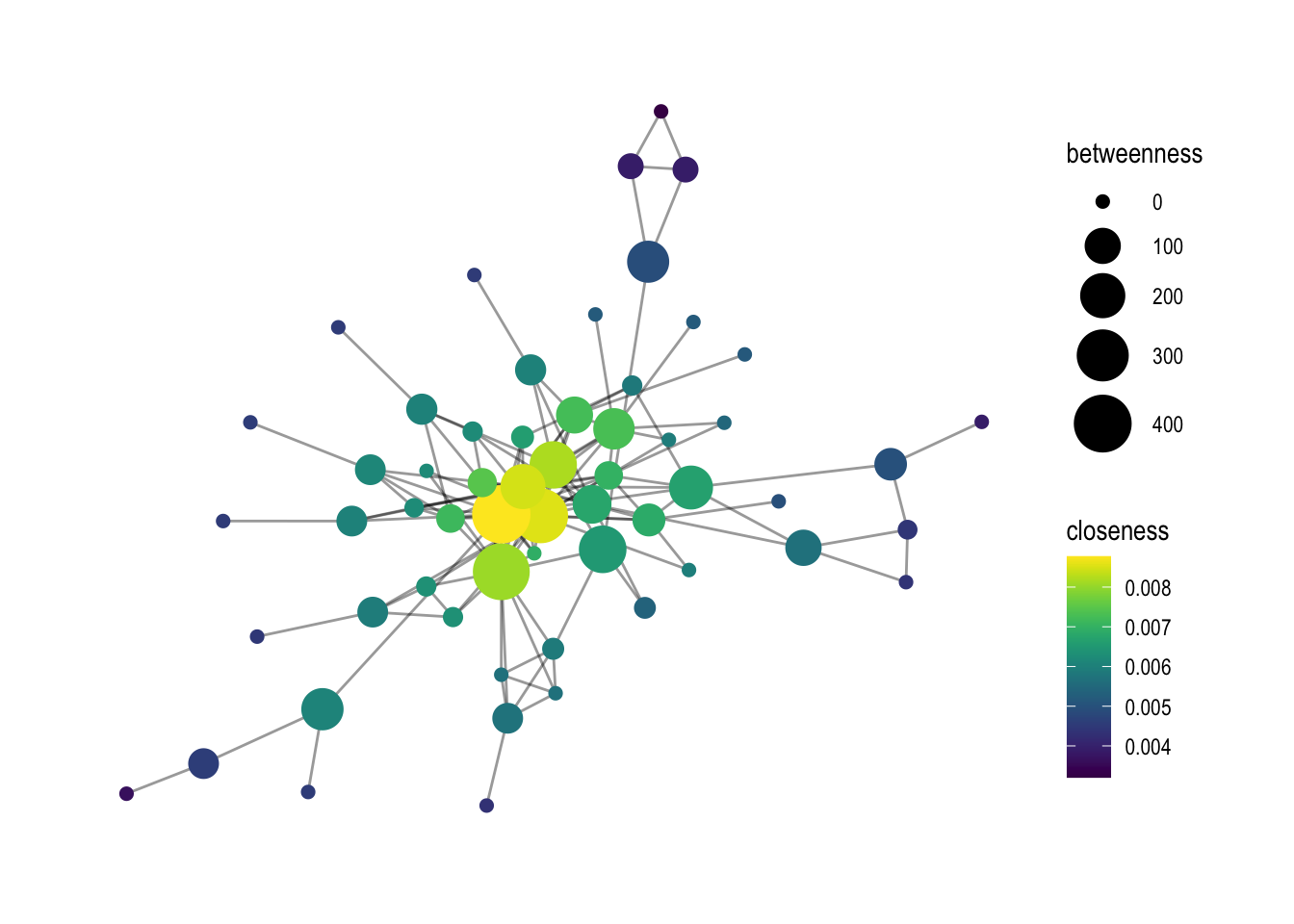``````# otherwise create it yourself, colors can be chosen by looking at colors()
p1 + scale_color_gradient2(low='white', mid='wheat',  high='mediumvioletred', na.value='green')
``````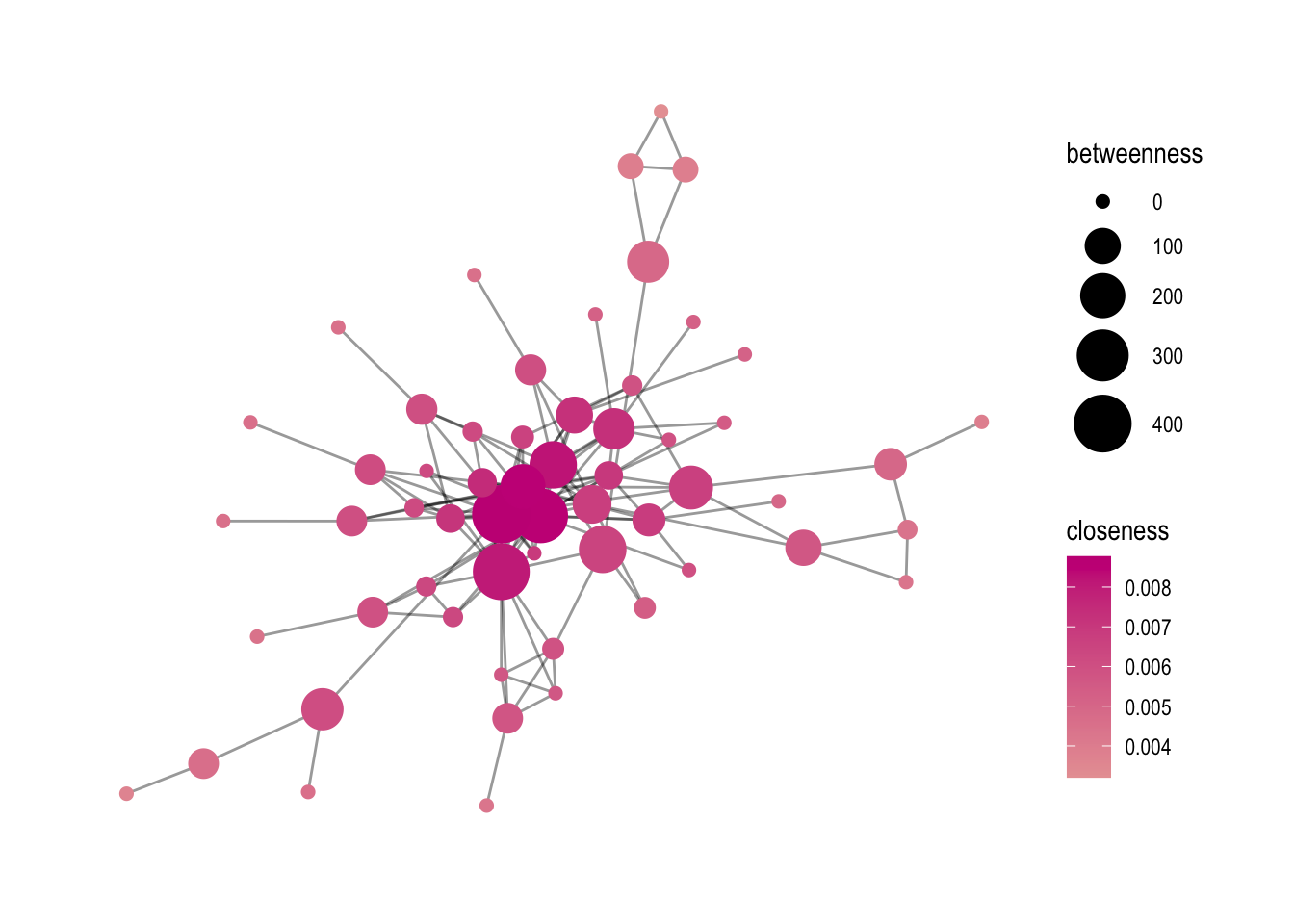Finally, the `ggsave` function is used to save the graph as a PNG file. The parameters specify the name of the output file, the plot object (`p1`), and the dimensions of the plot in centimeters.

``````# save as png or as pdf
ggsave('output/elitedb-graph-lektion06_01.png', plot = p1, width=30, height=17.5, unit='cm')
``````

## 7.3 Analysis of centrality measures using ggplot2 #

Sometimes, it can be useful to further analyse the association between centrality measures. Here, I am showing two options on how to analyze their interaction.

First, I create a data frame called `metrics` using the `tibble()` function. This data frame contains various centrality measures for the nodes in the graph, such as degree, betweenness, closeness, eigen centrality, and brokerage. These measures are calculated using functions such as `degree()`, `betweenness()`, `closeness()`, `eigen_centrality()`, and `constraint()`.

``````metrics <- tibble(
name = names(degree(comp1, mode="all")),
degree =        degree(comp1, mode="all"),
betweenness =   betweenness(comp1, directed=FALSE, weights=NA),
closeness =     closeness(comp1, mode="all", weights=NA),
eigen =         eigen_centrality(comp1, directed=FALSE, weights=NA)\$vector,
brokerage= 1-constraint(comp1)
)
``````

In the data frame, I also want to include a column called `firmtype`, which will be used to categorize the nodes in the graph by their business type. To create this column, a vector called `list1` is defined that contains the names of various business types, such as “only_bank” and “bank_pension”. The `metrics` data frame is then updated using a for loop that assigns each node in the graph to its corresponding business type based on the node’s name.

``````#create a column with firmtypes
list1 <- c("only_bank", "only_finance", "only_pension", "bank_finance", "bank_pension", "finance_pension", "bank_finance_pension")

# make an empty column called firmtype
metrics\$firmtype <- NA

# loop through list1 and rename NAs with names in list1 by their location.
for(i in list1) {

metrics\$firmtype[which(metrics\$name %in% get(i))] <- i

}
``````

After creating the `metrics` data frame, two visualization options are provided using `ggplot2`.

• Option 1: A scatter plot of the “brokerage” centrality measure on the x-axis and the “eigen” centrality measure on the y-axis, with the size of the points representing the “closeness” centrality measure and the color of the points representing the “firmtype”.
``````# What can you visualize: Option 1
metrics %>%
ggplot(aes(x = brokerage, y = eigen, color=firmtype, size=closeness)) +
geom_point() +
scale_color_manual(values=my_colors)
``````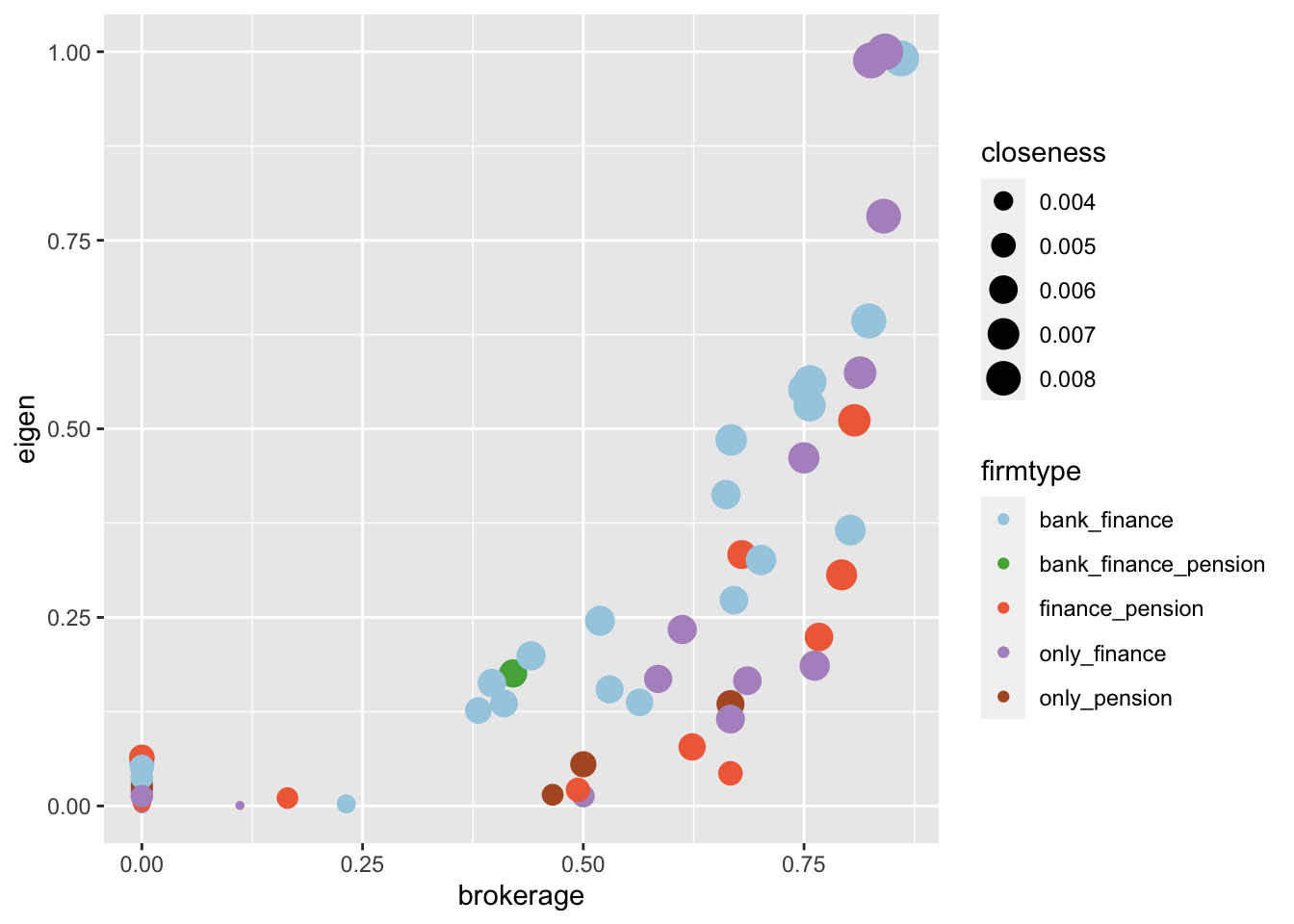• Option 2: A histogram of the “degree” centrality measure for each firm type, with each firm type represented by a different colored bar. The facet_wrap function allows us to separate the histograms by firm type.
``````# Option2
metrics %>%
ggplot(aes(x = degree, fill=firmtype)) + geom_histogram(binwidth=1) +
labs(y='amount') +
theme_minimal() +
facet_wrap(~firmtype) +
scale_fill_manual(values=my_colors)
``````